# Existence of solutions for a class of biharmonic equations with the Navier boundary value condition

## Abstract

In this paper, the existence of at least one nontrivial solution for a class of fourth-order elliptic equations with the Navier boundary value conditions is established by using the linking methods.

## 1 Introduction

Consider the following Navier boundary value problem:

(1.1)

where ${\mathrm{△}}^{2}$ is the biharmonic operator, $l\in R$ and Ω is a bounded smooth domain in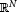($N>4$).

The conditions imposed on $f\left(x,t\right)$ are as follows:

(H1)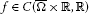, and there are constants ${C}_{1},{C}_{2}\ge 0$ such that

$|f\left(x,t\right)|\le {C}_{1}+{C}_{2}{|t|}^{s-1},\phantom{\rule{1em}{0ex}}\mathrm{\forall }x\in \mathrm{\Omega },\mathrm{\forall }t\in R,s\in \left(2,{p}^{\ast }\right)\left(N>4\right),$

where ${p}^{\ast }=\frac{2N}{N-4}$;

(H2) $f\left(x,t\right)=\circ \left(|t|\right)$, $|t|\to 0$, uniformly on Ω;

(H3) ${lim}_{|t|\to \mathrm{\infty }}\frac{f\left(x,t\right)}{t}=+\mathrm{\infty }$ uniformly on Ω;

(H4) There is a constant $\theta \ge 1$ such that for all $\left(x,t\right)\in \mathrm{\Omega }×R$ and $s\in \left[0,1\right]$,

$\theta \left(f\left(x,t\right)t-2F\left(x,t\right)\right)\ge \left(sf\left(x,st\right)t-2F\left(x,st\right)\right),$

where $F\left(x,t\right)={\int }_{0}^{t}f\left(x,s\right)\phantom{\rule{0.2em}{0ex}}ds$;

(H5) For some $\delta >0$, either

or

This fourth-order semilinear elliptic problem has been studied by many authors. In , there was a survey of results obtained in this direction. In , Micheletti and Pistoia showed that (1.1) admits at least two solutions by a variation of linking if $f\left(x,u\right)$ is sublinear. And in , the authors proved that the problem (1.1) has at least three solutions by a variational reduction method and a degree argument. In , Zhang and Li showed that (1.1) admits at least two nontrivial solutions by the Morse theory and local linking if $f\left(x,u\right)$ is superlinear and subcritical on u. In , Zhang and Wei obtained the existence of infinitely many solutions for the problem (1.1) where the nonlinearity involves a combination of superlinear and asymptotically linear terms. As far as the problem (1.1) is concerned, existence results of sign-changing solutions were also obtained. See, e.g., [6, 7] and the references therein.

We will use linking methods to give the existence of at least one nontrivial solution for (1.1).

Let X be a Banach space with a direct sum decomposition

$X={X}^{1}\oplus {X}^{2}.$

The function $I\in {C}^{1}\left(X,R\right)$ has a local linking at 0, with respect to $\left({X}^{1},{X}^{2}\right)$ if for some $r>0$,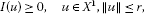(1.2)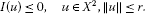(1.3)

It is clear that 0 is a critical point of I.

The notion of local linking generalizes the notions of local minimum and local maximum. When 0 is a non-degenerate critical point of a functional of class ${C}^{2}$ defined on a Hilbert space and $I\left(0\right)=0$, I has local linking at 0.

The condition of local linking was introduced in  under stronger assumptions

$I\left(u\right)\ge c>0,\phantom{\rule{1em}{0ex}}u\in {X}^{1},\parallel u\parallel =r,dim{X}^{2}<\mathrm{\infty }.$

Under those assumptions, the existence of nontrivial critical points was proved for functionals which are

1. (a)

bounded below ,

2. (b)

3. (c)

The cases (a), (b) and (c) were considered in  with only conditions (1.2) and (1.3), and three theorems about critical points were proved. Using these theorems, the author in  proved the existence of at least one nontrivial solution for the second-order elliptic boundary value problem with the Dirichlet boundary value condition. In the present paper, we also use the three theorems in  and the linking technique to give the existence of at least one nontrivial solution for the biharmonic problem (1.1) under a weaker condition. The main results are as follows.

Theorem 1.1 Assume the conditions (H1)-(H4) hold. If l is an eigenvalue of (with the Dirichlet boundary condition), assume also (H5). Then the problem (1.1) has at least one nontrivial solution.

We also consider asymptotically quadratic functions.

Let $0<{\lambda }_{1}<{\lambda }_{2}<\cdots <{\lambda }_{k}<\cdots$ be the eigenvalues of $\left(-\mathrm{△},{H}_{0}^{1}\left(\mathrm{\Omega }\right)\right)$. Then ${\mu }_{j}$ ($j\in {N}_{+}$) is the eigenvalue of $\left({\mathrm{△}}^{2}+l\mathrm{△},{H}^{2}\left(\mathrm{\Omega }\right)\cap {H}_{0}^{1}\left(\mathrm{\Omega }\right)\right)$, where ${\mu }_{j}={\lambda }_{j}\left({\lambda }_{j}-l\right)$. We assume that

(H6) $f\left(x,u\right)={f}_{\mathrm{\infty }}u+\circ \left(|u|\right)$, $|u|\to \mathrm{\infty }$, uniformly in Ω, and ${\mu }_{k}<{f}_{\mathrm{\infty }}<{\mu }_{k+1}$.

Theorem 1.2 Assume the conditions (H1), (H6) and one of the following conditions:

(A1) ${\lambda }_{j}, $j\ne k$;

(A2) ${\lambda }_{j}=l<{\lambda }_{j+1}$, $j\ne k$, for some $\delta >0$,

$F\left(x,u\right)\ge 0,\phantom{\rule{1em}{0ex}}\mathit{\text{for}}|u|>\delta ,x\in \mathrm{\Omega };$

(A3) ${\lambda }_{j}, $j\ne k$, for some $\delta >0$,

$F\left(x,u\right)\ge 0,\phantom{\rule{1em}{0ex}}\mathit{\text{for}}|u|\le \delta ,x\in \mathrm{\Omega }.$

Then the problem (1.1) has at least one nontrivial solution.

## 2 Preliminaries

Let X be a Banach space with a direct sum decomposition

$X={X}^{1}\oplus {X}^{2}.$

Consider two sequences of a subspace:

${X}_{0}^{1}\subset {X}_{1}^{1}\subset \cdots \subset {X}^{1},\phantom{\rule{2em}{0ex}}{X}_{0}^{2}\subset {X}_{1}^{2}\subset \cdots \subset {X}^{2}$

such that

${X}^{j}=\bigcup _{n\in N}{X}_{n}^{j},\phantom{\rule{1em}{0ex}}j=1,2.$

For every multi-index $\alpha =\left({\alpha }_{1},{\alpha }_{2}\right)\in {N}^{2}$, let ${X}_{\alpha }={X}_{{\alpha }_{1}}\oplus {X}_{{\alpha }_{2}}$. We know that

$\alpha \le \beta \phantom{\rule{1em}{0ex}}⇔\phantom{\rule{1em}{0ex}}{\alpha }_{1}\le {\beta }_{1},\phantom{\rule{2em}{0ex}}{\alpha }_{2}\le {\beta }_{2}.$

A sequence $\left({\alpha }_{n}\right)\subset {N}^{2}$ is admissible if for every $\alpha \in {N}^{2}$, there is $m\in N$ such that $n\ge m⇒{\alpha }_{n}\ge \alpha$. For every $I:X\to R$, we denote by ${I}_{\alpha }$ the function I restricted ${X}_{\alpha }$.

Definition 2.1 Let I be locally Lipschitz on X and $c\in R$. The functional I satisfies the ${\left(C\right)}_{c}^{\ast }$ condition if every sequence $\left({u}_{{\alpha }_{n}}\right)$ such that $\left({\alpha }_{n}\right)$ is admissible and

${u}_{{\alpha }_{n}}\in {X}_{{\alpha }_{n}},\phantom{\rule{1em}{0ex}}I\left({u}_{{\alpha }_{n}}\right)\to c,\phantom{\rule{2em}{0ex}}\left(1+\parallel {u}_{{\alpha }_{n}}\parallel \right){I}^{\prime }\left({u}_{{\alpha }_{n}}\right)\to 0$

contains a subsequence which converges to a critical point of I.

Definition 2.2 Let I be locally Lipschitz on X and $c\in R$. The functional I satisfies the ${\left(C\right)}^{\ast }$ condition if every sequence $\left({u}_{{\alpha }_{n}}\right)$ such that $\left({\alpha }_{n}\right)$ is admissible and

${u}_{{\alpha }_{n}}\in {X}_{{\alpha }_{n}},\phantom{\rule{1em}{0ex}}\underset{n}{sup}I\left({u}_{{\alpha }_{n}}\right)\le c,\phantom{\rule{2em}{0ex}}\left(1+\parallel {u}_{{\alpha }_{n}}\parallel \right){I}^{\prime }\left({u}_{{\alpha }_{n}}\right)\to 0$

contains a subsequence which converges to a critical point of I.

Remark 2.1

1. 1.

The ${\left(C\right)}^{\ast }$ condition implies the ${\left(C\right)}_{c}^{\ast }$ condition for every $c\in R$.

2. 2.

When the ${\left(C\right)}_{c}^{\ast }$ sequence is bounded, then the sequence is a ${\left(PS\right)}_{c}^{\ast }$ sequence (see ).

3. 3.

Without loss of generality, we assume that the norm in X satisfies

${\parallel {u}_{1}+{u}_{2}\parallel }^{2}={\parallel {u}_{1}\parallel }^{2}+{\parallel {u}_{2}\parallel }^{2},\phantom{\rule{1em}{0ex}}{u}_{j}\in {X}_{j},j=1,2.$

Definition 2.3 Let X be a Banach space with a direct sum decomposition

$X={X}_{1}\oplus {X}_{2}.$

The function $I\in {C}^{1}\left(X,R\right)$ has a local linking at 0, with respect to $\left({X}^{1},{X}^{2}\right)$, if for some $r>0$,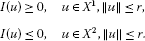Lemma 2.1 (see )

Suppose that $I\in {C}^{1}\left(X,R\right)$ satisfies the following assumptions:

(B1) I has a local linking at 0 and ${X}^{1}\ne \left\{0\right\}$;

(B2) I satisfies ${\left(PS\right)}^{\ast }$;

(B3) I maps bounded sets into bounded sets;

(B4) for every $m\in N$, $I\left(u\right)\to -\mathrm{\infty }$, $\parallel u\parallel \to \mathrm{\infty }$, $u\in X={X}_{m}^{1}\oplus {X}^{2}$. Then I has at least two critical points.

Remark 2.2 Assume I satisfies the ${\left(C\right)}_{c}^{\ast }$ condition. Then this theorem still holds.

Let X be a real Hilbert space and let $I\in {C}^{1}\left(X,R\right)$. The gradient of I has the form

$\mathrm{\nabla }I\left(u\right)=Au+B\left(u\right),$

where A is a bounded self-adjoint operator, 0 is not the essential spectrum of A, and B is a nonlinear compact mapping.

We assume that there exist an orthogonal decomposition,

$X={X}_{1}+{X}_{2},$

and two sequences of finite-dimensional subspaces,

${X}_{0}^{1}\subset {X}_{1}^{1}\subset {X}_{1}^{1}\subset \cdots \subset {X}^{1},\phantom{\rule{2em}{0ex}}{X}_{0}^{2}\subset {X}_{1}^{2}\subset \cdots \subset {X}^{2},$

such that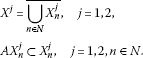For every multi-index $\alpha =\left({\alpha }_{1},{\alpha }_{2}\right)\in {N}^{2}$, we denote by ${X}_{\alpha }$ the space

${X}_{\alpha }^{1}\oplus {X}_{\alpha }^{2},$

by ${p}_{\alpha }:X\to {X}_{\alpha }$ the orthogonal projector onto ${X}_{\alpha }$, and by ${M}^{-}\left(L\right)$ the Morse index of a self-adjoint operator L.

Lemma 2.2 (see )

I satisfies the following assumptions:

1. (i)

I has a local linking at 0 with respect to $\left({X}^{1},{X}^{2}\right)$;

2. (ii)

there exists a compact self-adjoint operator ${B}_{\mathrm{\infty }}$ such that

$B\left(u\right)={B}_{\mathrm{\infty }}\left(u\right)+\circ \left(\parallel u\parallel \right),\phantom{\rule{1em}{0ex}}\parallel u\parallel \to \mathrm{\infty };$
3. (iii)

$A+{B}_{\mathrm{\infty }}$ is invertible;

4. (iv)

for infinitely many multiple-indices $\alpha :=\left(n,n\right)$,

${M}^{-}\left(\left(A+{P}_{\alpha }{B}_{\mathrm{\infty }}\right){|}_{{X}_{\alpha }}\right)\ne dim{X}_{n}^{2}.$

Then I has at least two critical points.

## 3 The proof of main results

Proof of Theorem 1.1 (1) We shall apply Lemma 2.1 to the functional

$I\left(u\right)=\frac{1}{2}{\int }_{\mathrm{\Omega }}\left({|\mathrm{△}u|}^{2}-l{|\mathrm{\nabla }u|}^{2}\right)\phantom{\rule{0.2em}{0ex}}dx-{\int }_{\mathrm{\Omega }}F\left(x,u\right)\phantom{\rule{0.2em}{0ex}}dx$

defined on $X={H}_{0}^{1}\left(\mathrm{\Omega }\right)\cap {H}^{2}\left(\mathrm{\Omega }\right)$. We consider only the case $l={\lambda }_{j}$, and

(3.1)

Then other case is similar and simple.

Let ${X}^{2}$ be the finite dimensional space spanned by the eigenfunctions corresponding to negative eigenvalues of $-{\mathrm{△}}^{2}+l\mathrm{△}$ and let ${X}^{1}$ be its orthogonal complement in X. Choose a Hilbertian basis ${e}_{n}$ ($n\ge 0$) for X and define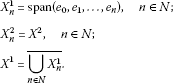By the condition (H1) and Sobolev inequalities, it is easy to see that the functional I belongs to ${C}^{1}\left(X,R\right)$ and maps bounded sets to bounded sets.

(2) We claim that I has a local linking at 0 with respect to $\left({X}^{1},{X}^{2}\right)$. Decompose ${X}^{1}$ into $V+W$ when $V=ker\left(-{\mathrm{△}}^{2}+l\mathrm{△}\right)$, $W={\left({X}^{2}+V\right)}^{\mathrm{\perp }}$. Also, set $u=v+w$, $u\in {X}^{1}$, $v\in V$, $w\in W$. By the equivalence of norm in the finite-dimensional space, there exists $C>0$ such that

${\parallel v\parallel }_{\mathrm{\infty }}\le C{\parallel v\parallel }_{X},\phantom{\rule{1em}{0ex}}\mathrm{\forall }v\in V.$
(3.2)

It follows from (H1) and (H2) that for any $ϵ>0$, there exists ${C}_{ϵ}$ such that

$|F\left(x,u\right)|\le ϵ{u}^{2}+{C}_{ϵ}{|u|}^{s}.$
(3.3)

Hence, we obtain

$\begin{array}{rcl}I\left(u\right)& \le & \frac{1}{2}{\int }_{\mathrm{\Omega }}\left({|\mathrm{△}u|}^{2}-l{|\mathrm{\nabla }u|}^{2}\right)\phantom{\rule{0.2em}{0ex}}dx+ϵ{\int }_{\mathrm{\Omega }}{u}^{2}\phantom{\rule{0.2em}{0ex}}dx+c{\parallel u\parallel }_{X}^{s+1}\\ \le & -m{\parallel u\parallel }^{2}+ϵ{\int }_{\mathrm{\Omega }}{u}^{2}\phantom{\rule{0.2em}{0ex}}dx+{c}^{\ast }{\parallel u\parallel }_{X}^{s+1},\end{array}$

where $m>0$, ${c}^{\ast }$ is a constant and hence, for $r>0$ small enough,

$I\left(u\right)\le 0,\phantom{\rule{1em}{0ex}}u\in {X}^{2},{\parallel u\parallel }_{X}\le r.$

Let $u=v+w\in {X}^{1}$ be such that ${\parallel u\parallel }_{X}\le {r}_{1}=\frac{\delta }{2C}$ and let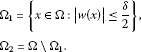From (3.2), we have

$|v\left(x\right)|\le {\parallel v\parallel }_{\mathrm{\infty }}\le C\parallel v\parallel \le \frac{\delta }{2}$

for all $\parallel u\parallel \le {r}_{1}$ and $x\in \mathrm{\Omega }$. On the one hand, one has $|u\left(x\right)|\le |v\left(x\right)|+|w\left(x\right)|\le {\parallel v\parallel }_{\mathrm{\infty }}+\frac{\delta }{2}\le \delta$ for all $x\in {\mathrm{\Omega }}_{1}$. Hence, from (H5), we obtain

${\int }_{{\mathrm{\Omega }}_{1}}F\left(x,u\right)\phantom{\rule{0.2em}{0ex}}dx\le 0.$

On the other hand, we have

$|u\left(x\right)|\le |v\left(x\right)|+|w\left(x\right)|\le \frac{\delta }{2}+|w\left(x\right)|\le 2|w\left(x\right)|$

for all $x\in {\mathrm{\Omega }}_{2}$. It follows from (3.3) that

$F\left(x,u\right)\le ϵ{u}^{2}+{C}_{ϵ}{|u|}^{s+1}\le 4ϵ{w}^{2}+{2}^{s+1}{C}_{ϵ}{|w|}^{s+1}$

for all $x\in {\mathrm{\Omega }}_{2}$ and all $u\in {X}_{1}$ with $\parallel u\parallel \le {r}_{1}$, which implies that

$\begin{array}{rcl}{\int }_{\mathrm{\Omega }}F\left(x,u\right)\phantom{\rule{0.2em}{0ex}}dx& \le & 4ϵ{\int }_{{\mathrm{\Omega }}_{2}}{w}^{2}\phantom{\rule{0.2em}{0ex}}dx+{\int }_{{\mathrm{\Omega }}_{2}}{2}^{s+1}{C}_{ϵ}{|w|}^{s+1}\phantom{\rule{0.2em}{0ex}}dx\\ \le & 4{\left({C}_{3}\right)}^{2}ϵ{\parallel w\parallel }^{2}+{\left(2{C}_{3}\right)}^{\lambda +1}{C}_{ϵ}{\parallel w\parallel }^{s+1},\end{array}$

where ${C}_{3}$ is a constant. Hence, there exist positive constants ${C}^{\ast \ast }$, ${C}_{4}$ and ${C}_{5}$ such that

$\begin{array}{rcl}I\left(u\right)& =& \frac{1}{2}{\parallel w\parallel }^{2}-\frac{1}{2}{\int }_{\mathrm{\Omega }}l{|\mathrm{\nabla }w|}^{2}\phantom{\rule{0.2em}{0ex}}dx-{\int }_{{\mathrm{\Omega }}_{2}}F\left(x,u\right)\phantom{\rule{0.2em}{0ex}}dx-{\int }_{{\mathrm{\Omega }}_{1}}F\left(x,u\right)\phantom{\rule{0.2em}{0ex}}dx\\ \ge & {C}^{\ast \ast }{\parallel w\parallel }^{2}-4{\left({C}_{3}\right)}^{2}ϵ{\parallel w\parallel }^{2}-{\left(2{C}_{3}\right)}^{\lambda +1}{C}_{ϵ}{\parallel w\parallel }^{s+1}-{\int }_{{\mathrm{\Omega }}_{1}}G\left(x,u\right)\phantom{\rule{0.2em}{0ex}}dx\\ \ge & {C}_{4}{\parallel w\parallel }^{2}-{C}_{5}{\parallel w\parallel }^{s+1}\end{array}$

for all $u\in {X}^{1}$ with $\parallel u\parallel \le {r}_{1}$, which implies that

for $0 small enough.

(3) We claim that I satisfies ${\left(C\right)}_{c}^{\ast }$. Consider a sequence $\left({u}_{{\alpha }_{n}}\right)$ such that $\left({u}_{{\alpha }_{n}}\right)$ is admissible and

${u}_{{\alpha }_{n}}\in {X}_{{\alpha }_{n}},\phantom{\rule{1em}{0ex}}I\left({u}_{{\alpha }_{n}}\right)\to c,\phantom{\rule{2em}{0ex}}\left(1+\parallel {u}_{{\alpha }_{n}}\parallel \right){I}^{\prime }\left({u}_{{\alpha }_{n}}\right)\to 0$
(3.4)

and

$\underset{n\to \mathrm{\infty }}{lim}{\int }_{\mathrm{\Omega }}\left(\frac{1}{2}f\left(x,{u}_{{\alpha }_{n}}\right){u}_{{\alpha }_{n}}-F\left(x,{u}_{{\alpha }_{n}}\right)\right)\phantom{\rule{0.2em}{0ex}}dx=c.$
(3.5)

Let ${w}_{{\alpha }_{n}}={\parallel {u}_{{\alpha }_{n}}\parallel }^{-1}{u}_{{\alpha }_{n}}$. Up to a subsequence, we have

If $w=0$, we choose a sequence $\left\{{t}_{n}\right\}\subset \left[0,1\right]$ such that

$I\left({t}_{n}{u}_{{\alpha }_{n}}\right)=\underset{t\in \left[0,1\right]}{max}I\left(t{u}_{{\alpha }_{n}}\right).$

For any $m>0$, let ${v}_{{\alpha }_{n}}=2\sqrt{m}{w}_{{\alpha }_{n}}$. By the Sobolev imbedded theory, we have

$\underset{n\to \mathrm{\infty }}{lim}{\int }_{\mathrm{\Omega }}F\left(x,{v}_{{\alpha }_{n}}\right)\phantom{\rule{0.2em}{0ex}}dx=0.$

So, for n large enough, $2\sqrt{m}{\parallel {u}_{{\alpha }_{n}}\parallel }^{-1}\in \left(0,1\right)$, and combining Ehrling-Nirenberg-Gagliardo inequality, we have

$I\left({t}_{n}{u}_{{\alpha }_{n}}\right)\ge I\left({v}_{{\alpha }_{n}}\right)\ge m-ϵ\ge \frac{m}{2},$
(3.6)

where ϵ is a small enough constant.

That is, $I\left({t}_{n}{u}_{{\alpha }_{n}}\right)\to \mathrm{\infty }$. Now, $I\left(0\right)=0$, $I\left({u}_{{\alpha }_{n}}\right)\to c$, we know that ${t}_{n}\in \left[0,1\right]$ and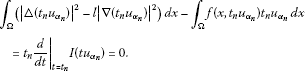(3.7)

Therefore, using (H3), we have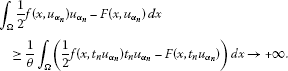If $w\ne 0$, then the set $⊝=\left\{x\in \mathrm{\Omega }:w\left(x\right)\ne 0\right\}$ has a positive Lebesgue measure. For $x\in ⊝$, we have $|{u}_{{\alpha }_{n}}\left(x\right)|\to \mathrm{\infty }$. Hence, by (H3), we have

$\frac{f\left(x,{u}_{{\alpha }_{n}}\left(x\right)\right){u}_{{\alpha }_{n}}\left(x\right)}{{|{u}_{{\alpha }_{n}}\left(x\right)|}^{2}}{|{w}_{{\alpha }_{n}}\left(x\right)|}^{2}\phantom{\rule{0.2em}{0ex}}dx\to \mathrm{\infty }.$
(3.8)

From (3.4), we obtain

$1-\circ \left(1\right)\ge \left({\int }_{w\ne 0}+{\int }_{w=0}\right)\frac{f\left(x,{u}_{{\alpha }_{n}}\left(x\right)\right){u}_{{\alpha }_{n}}\left(x\right)}{{|{u}_{{\alpha }_{n}}\left(x\right)|}^{2}}{|{w}_{{\alpha }_{n}}\left(x\right)|}^{2}\phantom{\rule{0.2em}{0ex}}dx.$
(3.9)

By (3.8), the right-hand side of (3.9) $\to +\mathrm{\infty }$. This is a contradiction.

In any case, we obtain a contradiction. Therefore, $\left\{{u}_{{\alpha }_{n}}\right\}$ is bounded.

Finally, we claim that for every $m\in N$,

By (H2) and (H3), there exists large enough M such that

$F\left(x,t\right)\ge M{t}^{2}-{C}_{6},\phantom{\rule{1em}{0ex}}x\in \mathrm{\Omega },t\in \mathbb{R}.$

So, for any $u\in {X}_{m}^{1}\oplus {X}^{2}$, we have

Hence, our claim holds. □

Proof of Theorem 1.2 We omit the proof which depends on Lemma 2.2 and is similar to the preceding one. □

## Author’s contributions

The author read and approved the final manuscript.

## References

1. Lazer AC, Mckenna PJ: Large amplitude periodic oscillation in suspension bridges: some new connections with nonlinear analysis. SIAM Rev. 1990, 32: 537-578. 10.1137/1032120

2. Micheletti AM, Pistoia A: Multiplicity solutions for a fourth order semilinear elliptic problems. Nonlinear Anal. TMA 1998, 31: 895-908. 10.1016/S0362-546X(97)00446-X

3. Chipot M: Variational Inequalities and Flow in Porous Media. Springer, New York; 1984.

4. Zhang JH, Li SJ: Multiple nontrivial solutions for some fourth-order semilinear elliptic problems. Nonlinear Anal. TMA 2005, 60: 221-230.

5. Zhang J, Wei ZL: Infinitely many nontrivial solutions for a class of biharmonic equations via variant fountain theorems. Nonlinear Anal. TMA 2011, 74: 7474-7485. 10.1016/j.na.2011.07.067

6. Zhou JW, Wu X: Sign-changing solutions for some fourth-order nonlinear elliptic problems. J. Math. Anal. Appl. 2008, 342: 542-558. 10.1016/j.jmaa.2007.12.020

7. Liu XQ, Huang YS: One sign-changing solution for a fourth-order asymptotically linear elliptic problem. Nonlinear Anal. TMA 2010, 72: 2271-2276. 10.1016/j.na.2009.11.001

8. Liu JQ, Li SJ: Some existence theorems on multiple critical points and their applications. Chin. Sci. Bull. 1984, 17: 1025-1027.

9. Li SJ, Liu JQ: Morse theory and asymptotic linear Hamiltonian systems. J. Differ. Equ. 1989, 78: 53-73. 10.1016/0022-0396(89)90075-2

10. Li SJ, Willem M: Applications of local linking to critical point theory. J. Math. Anal. Appl. 1995, 189: 6-32. 10.1006/jmaa.1995.1002

11. Teng KM: Existence and multiplicity results for some elliptic systems with discontinuous nonlinearities. Nonlinear Anal. TMA 2012, 75: 2975-2987. 10.1016/j.na.2011.11.040

## Acknowledgements

The author would like to thank the referees for valuable comments and suggestions in improving this paper. This work was supported by the National NSF (Grant No. 10671156) of China.

## Author information

Authors

### Corresponding author

Correspondence to Ruichang Pei.

### Competing interests

The author declares that he has no competing interests.

## Rights and permissions

Reprints and Permissions

Pei, R. Existence of solutions for a class of biharmonic equations with the Navier boundary value condition. Bound Value Probl 2012, 154 (2012). https://doi.org/10.1186/1687-2770-2012-154